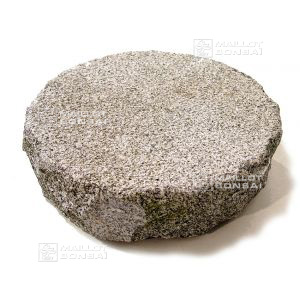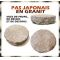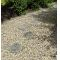##### The Japanese Bonsai specialist
Direct order Contact Help / Services Newsletter# Japanese stepping stones ø 30 cm granite

Pas japonais granit- tobi ishiref. : 4759

17,00

voluminous/heavy item extra shipping of 6,00

Available quantity : 22Order

###### Description

Diameter: 30 cm.

Weight: 13/15 kilograms.

Thickness: 5/10 cm. Irregular-shaped white granite stepping stones create the illusion of lengthening small gardens and can be complemented with gravel or pebbles of contrasting colours.

Our garden elements (basins, granite lanterns, rocks and Japanese stepping stones) are generally stocked outside. Indeed the patina and the ancient aspect obtained (lichens and mosses) gives an extra character of authenticity, which is usually really appreciated in traditional Japanese garden art.

#japanese 4.8 #stepping 4.8 #granite 4.7 #stones 4.6 #lantern 2.7 #garden 2.6 #stone 2.5 #authenticity 2.2 #complemented 2.2 #lengthening 2.1

Formule
(( ROUND((CHAR_LENGTH(b.article_nom)-CHAR_LENGTH(REPLACE(b.article_nom, 'stepping', '')))/LENGTH('stepping')) + ROUND((CHAR_LENGTH(b.article_description)-CHAR_LENGTH(REPLACE(b.article_description, 'stepping', '')))/LENGTH('stepping')) ) * 4.8) + (( ROUND((CHAR_LENGTH(b.article_nom)-CHAR_LENGTH(REPLACE(b.article_nom, 'japanese', '')))/LENGTH('japanese')) + ROUND((CHAR_LENGTH(b.article_description)-CHAR_LENGTH(REPLACE(b.article_description, 'japanese', '')))/LENGTH('japanese')) ) * 4.8) + (( ROUND((CHAR_LENGTH(b.article_nom)-CHAR_LENGTH(REPLACE(b.article_nom, 'granite', '')))/LENGTH('granite')) + ROUND((CHAR_LENGTH(b.article_description)-CHAR_LENGTH(REPLACE(b.article_description, 'granite', '')))/LENGTH('granite')) ) * 4.7) + (( ROUND((CHAR_LENGTH(b.article_nom)-CHAR_LENGTH(REPLACE(b.article_nom, 'stones', '')))/LENGTH('stones')) + ROUND((CHAR_LENGTH(b.article_description)-CHAR_LENGTH(REPLACE(b.article_description, 'stones', '')))/LENGTH('stones')) ) * 4.6) + (( ROUND((CHAR_LENGTH(b.article_nom)-CHAR_LENGTH(REPLACE(b.article_nom, 'garden', '')))/LENGTH('garden')) + ROUND((CHAR_LENGTH(b.article_description)-CHAR_LENGTH(REPLACE(b.article_description, 'garden', '')))/LENGTH('garden')) ) * 2.6) + (( ROUND((CHAR_LENGTH(b.article_nom)-CHAR_LENGTH(REPLACE(b.article_nom, 'authenticity', '')))/LENGTH('authenticity')) + ROUND((CHAR_LENGTH(b.article_description)-CHAR_LENGTH(REPLACE(b.article_description, 'authenticity', '')))/LENGTH('authenticity')) ) * 2.2) + (( ROUND((CHAR_LENGTH(b.article_nom)-CHAR_LENGTH(REPLACE(b.article_nom, 'complemented', '')))/LENGTH('complemented')) + ROUND((CHAR_LENGTH(b.article_description)-CHAR_LENGTH(REPLACE(b.article_description, 'complemented', '')))/LENGTH('complemented')) ) * 2.2) + (( ROUND((CHAR_LENGTH(b.article_nom)-CHAR_LENGTH(REPLACE(b.article_nom, 'traditional', '')))/LENGTH('traditional')) + ROUND((CHAR_LENGTH(b.article_description)-CHAR_LENGTH(REPLACE(b.article_description, 'traditional', '')))/LENGTH('traditional')) ) * 2.1) + (( ROUND((CHAR_LENGTH(b.article_nom)-CHAR_LENGTH(REPLACE(b.article_nom, 'contrasting', '')))/LENGTH('contrasting')) + ROUND((CHAR_LENGTH(b.article_description)-CHAR_LENGTH(REPLACE(b.article_description, 'contrasting', '')))/LENGTH('contrasting')) ) * 2.1) + (( ROUND((CHAR_LENGTH(b.article_nom)-CHAR_LENGTH(REPLACE(b.article_nom, 'lengthening', '')))/LENGTH('lengthening')) + ROUND((CHAR_LENGTH(b.article_description)-CHAR_LENGTH(REPLACE(b.article_description, 'lengthening', '')))/LENGTH('lengthening')) ) * 2.1)

## Secure payment## Delivery

Our logistic partners :04 74 55 23 48
Pépinière MAILLOT-BONSAÏ
Le Bois Frazy
01990 RELEVANT - FRANCE
on appointment